Get instant live expert help with Excel or Google Sheets“My Excelchat expert helped me in less than 20 minutes, saving me what would have been 5 hours of work!”

#### Post your problem and you'll get expert help in seconds

Your message must be at least 40 characters
Our professional experts are available now. Your privacy is guaranteed.

# How to find percentage difference between two numbers in Excel

When working with Excel spreadsheets,we may be required to get the percentage difference between 2 numbers. Percentage difference is simply the difference between the original value and the new value, expressed as a percentage.

## Formula to get percentage difference

We can calculate the percentage change in Excel using the formula below

`% of Difference = (New Value – Old value)/Old value`

When we calculate percentage difference with formula in Excel, the answer we get is simply in number format. Usually, we multiply the result with 100 in order to get the percentage difference in numbers. However, this is not the case when we are trying to find percentage difference between two numbers. Excel has a built-in feature that automatically formats the result into Excel percentage difference.

## How the Excel difference formula works

When using the percentage difference formula to calculate the difference, it first starts by calculating the difference between the specified values. This is the actual change, where the original value is subtracted from the new value. After getting the diff, the result is divided by the original value, which is also referred to as the old value. We get a decimal value result.

For us to get the difference as a percentage, we have to format it using percentage number format first.

## Examples of how to calculate percentage difference between two numbers in Excel

### Example 1: Calculate percentage difference between two columns

Step 1: Prepare the data for which you want to find the percentage difference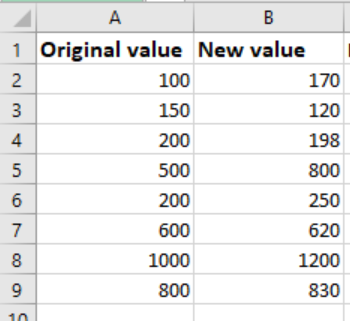Figure 1: Data to find difference

Step 2: Find the difference between two columns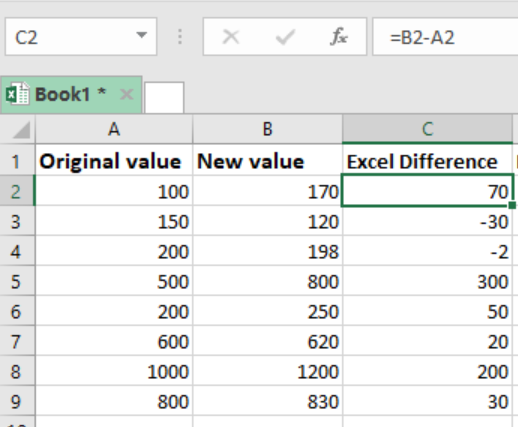Figure 2: Find the difference in numbers

Note how we find the difference in the above figure. All we need to do is subtract column B from A. in cell C2 for example, we have B2 – A2 to get 70 as the difference.

Step 3: Divide the difference with the original value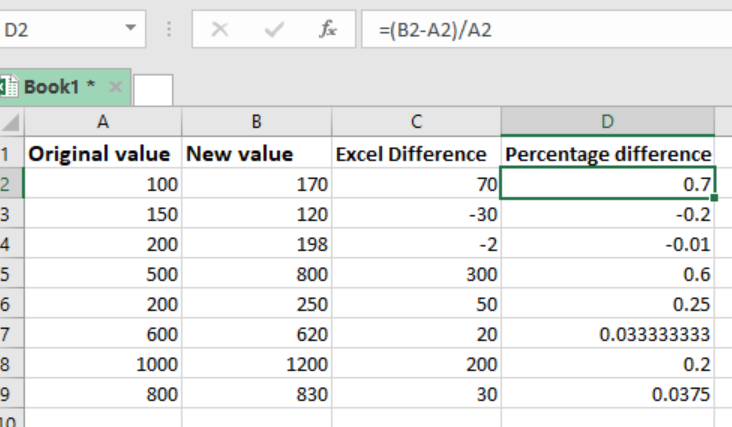Figure 3: Calculate percentage difference between original and new value

It is also important to note that when finding difference, we can have a positive difference or a negative difference. The positive differences are called increases while the negative differences are called decreases. In our figure above, you will notice that cell C3 and cell C4 have negative differences, meaning the change is negative.

In column D, we have the results in column C divided by the original value, also referred to as the old value to get the decimal results.

Step 4: Format to get the decimal results into percentage change

To format the difference column into a percentage, we need to first highlight it. Then after highlighting it, we right-click anywhere in the column to start formatting.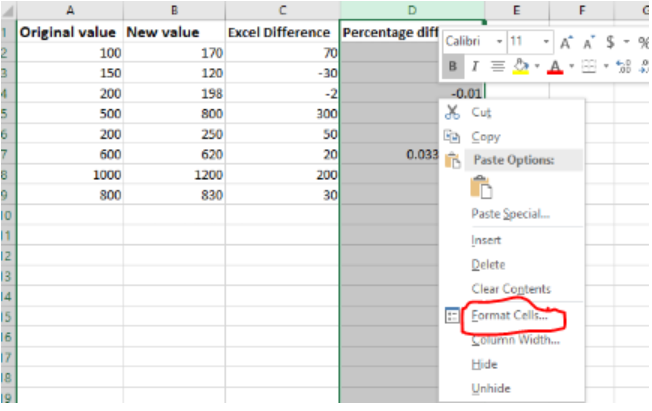Figure 4: Format to get the result as percentage change

In the dialog box that appears, choose numbers as your format type and the select percentage. Then click OK.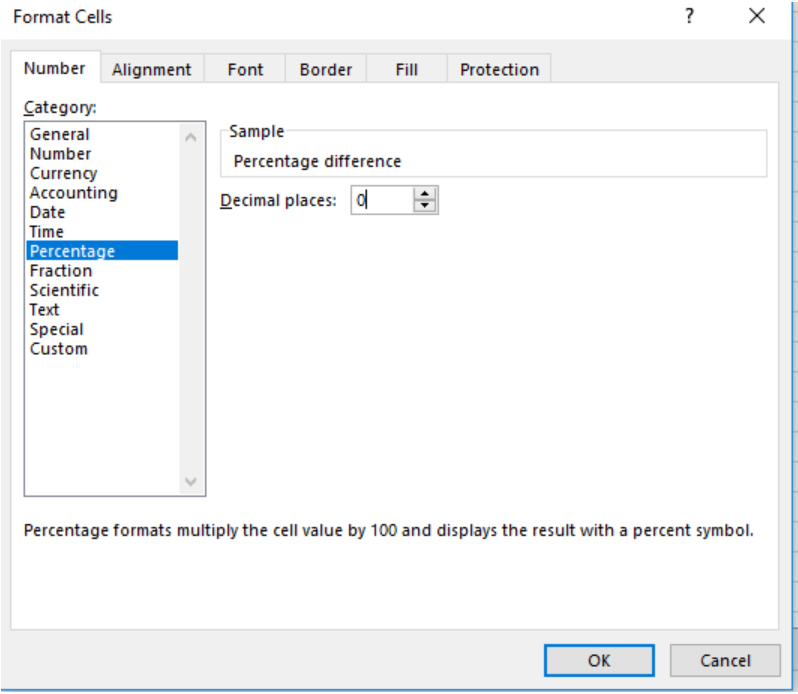Figure 5: Select Percentage as the format

After clicking on OK, you will have all the differences converted to percentage as shown in the figure below: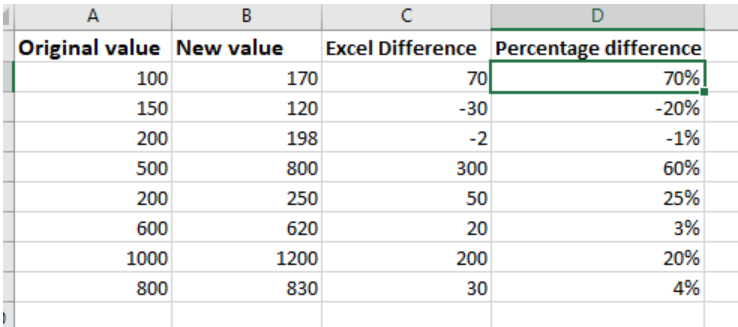Figure 6: Percentage difference

## NOTES

Perhaps, we should remember that we do not have a function for difference, thus we have to master how to use the difference formula to get changes between values.

## Instant Connection to an Expert through our Excelchat Service

Most of the time, the problem you will need to solve will be more complex than a simple application of a formula or function. If you want to save hours of research and frustration, try our live Excelchat service! Our Excel Experts are available 24/7 to answer any Excel question you may have. We guarantee a connection within 30 seconds and a customized solution within 20 minutes.